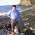## måndag 14 november 2011

### Heat Capacity of BlackbodyIn Mathematical Physics of Blackbody Radiation I derive an expression for the heat capacity C(T) of a blackbody in the form of a forced damped wave equation as function of temperature T, of the form
• C(T)= c T
with c a constant, thus with a linear dependence on T. This fits pretty well with observations for several solid metals for temperatures T smaller than 200 K, and for diamond up to 500 K, as shown above.

Recall that the theory of specific heats was studied intensely in the beginning of the 20th century: Einstein and Debye offered improvements for low temperatures of the classical Dulong-Petit Law C(T) = constant, using arguments from statistical mechanics.

I do not use statistical mechanics but instead a concept of finite precision computation.

#### 7 kommentarer:

1.Diamond at low temperatures goes as T^3, not linear in T. Your graph is false. Your citation (linked under "here") describes SiO2, not diamond, and that is also T^3, as the paper discusses.

T^3 is not linear in T. How do you explain it? It has been understood based on the nature of vibrations in solids for close to a century.

2.A free electron model has heat capacity prop to temp T, not T^3 as in the Einstein-Debye model.

3.I removed the ref to SiO2.

4.Yes, metals with free electrons means a 2-dimensional Fermi surface. That gives a linear-in-temperature low-temperature heat capacity, because the number of degrees of freedom grows linearly with energy near 0.

Diamond is *not* a metal, and its number of degrees of freedom has nothing to do with free electrons at low temperatures, rather it is purely atomic vibrations. The number of degrees of freedom grows as the cube of energy at low energies, just as the Debye model predicts. Your graph of diamond's heat capacity cannot be based on observations - do any literature search and you will see diamond's low-temperature heat capacity goes as T^3, not T.

5.The focus is not on very small temperatures where T^3 plays a role.

6.What is a small temperature then?

Heat capacity diamond

7.Here is another plot

http://www.scielo.org.ar/img/revistas/laar/v34n4/4a09g38.gif

showing heat capacity prop to T. My model is very basic and what is surprising is that it captures something of reality, but not everything.